## Linear models

“linear model” “general linear model” “linear predictor” “regression” “multiple regression” “multiple linear regression” “multiple linear regression model”

Learn to predict a response variable as

• a straight line relationship with a predictor variable
• or more than one predictor variable
• actually it doesn’t have to be a straight line
• some of the predictors could be categorical (ANOVA)
• and there could be interactions

• test which variables and interactions the data supports as predictors
• give a confidence interval on any estimates

## Linear models in R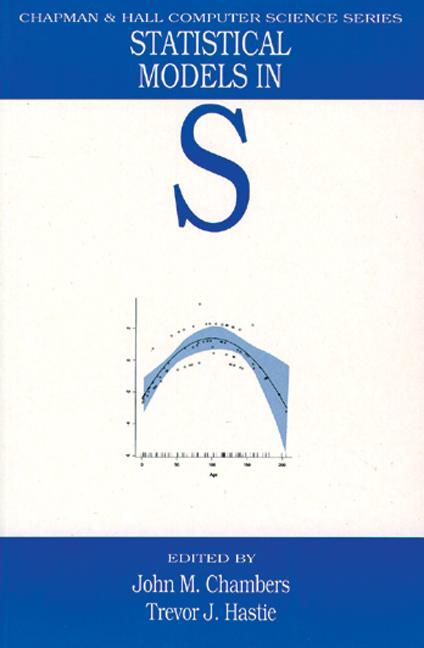Many features of the S language (predecessor to R) were created to support working with linear models and their generalizations:

• data.frame type introduced to hold data for modelling.
• factor type introduced to hold categorical data.
• y ~ x1+x2+x3 formula syntax specify terms in models.
• Manipulation of “S3” objects holding fitted models.
• Rich set of diagnostic visualization functions.

Primary reference is “Statistical models in S”.

## Linear maths and R

We will be using vectors and matrices extensively today.

In mathematics, we usually treat a vector as a matrix with a single column. In R, they are two different types. * R also makes a distinction between matrix and data.frame types. There is a good chance you have used data frames but not matrices before now.

Matrices contain all the same type of value, typically numeric, whereas data frames can have different types in different columns.

A matrix can be created from a vector using matrix, or from multiple vectors with cbind (bind columns) or rbind (bind rows), or from a data frame with as.matrix.

Matrix transpose exchanges rows and columns. In maths it is indicated with a small t, eg $$X^\top$$. In R use the t function, eg t(X).

$X = \begin{bmatrix} 1 & 4 \\ 2 & 5 \\ 3 & 6 \end{bmatrix} \quad \quad X^\top = \begin{bmatrix} 1 & 2 & 3 \\ 4 & 5 & 6 \end{bmatrix}$

## Linear maths and R

Taking the dot product of two vectors we multiply corresponding elements together and add the results to obtain a total.

In mathematical notation:

$a^\top b = \sum_{i=1}^{n} a_i b_i$

In R:

sum(a*b)

## Linear maths and R

Taking the product of a matrix $$X$$ and a vector $$a$$ with length matching the number of columns, the result is a vector containing the dot product of each row of the matrix $$X$$ with the vector $$a$$.

Can also think of this as a weighted sum of the columns of $$X$$.

$Xa = a_1 \begin{bmatrix} x_{1,1} \\ x_{2,1} \\ \vdots \end{bmatrix} + a_2 \begin{bmatrix} x_{1,2} \\ x_{2,2} \\ \vdots \end{bmatrix} + \dots$

In R:

X %*% a

(It’s also possible to multiply two matrices with %*% but we won’t need this today.)

## Geometry

The dot product is our ruler and set-square.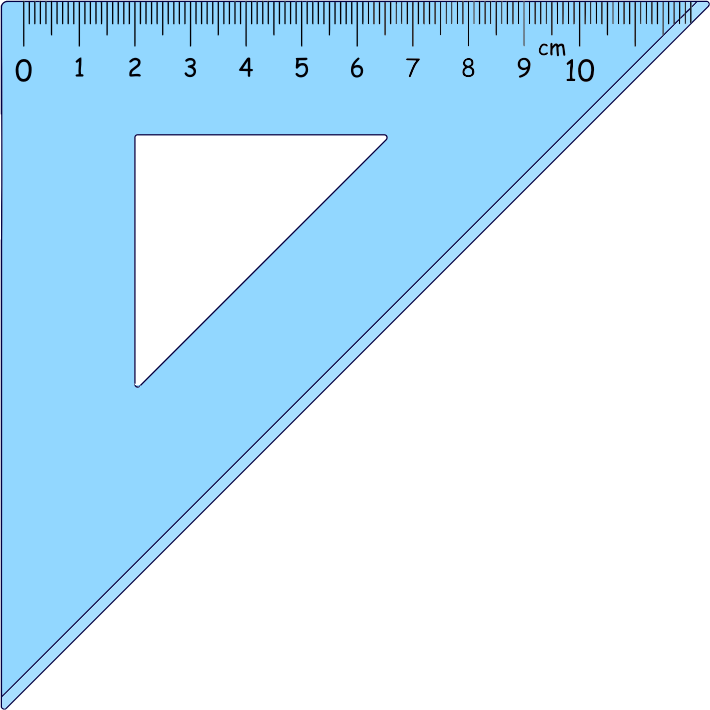### Lengths

A vector can be thought of as an arrow in a space.

The dot product of a vector with itself $$a^\top a$$ is the square of its length. Pythagorus!

### Right angles

Two vectors at right angles have a dot product of zero. They are orthogonal.

## Geometry - subspaces

• A line in two or more dimensions, passing through the origin.
• A plane in three or more dimensions, passing through the origin.
• A point at the origin.

These are all examples of subspaces.

Think of all the vectors that could result from multiplying a matrix $$X$$ with some arbitrary vector.

If the matrix $$X$$ has $$n$$ rows and $$p$$ columns, we obtain an (at most) $$p$$-dimensional subspace within an $$n$$-dimensional space.

A subspace has an orthogonal subspace with $$n-p$$ dimensions. All the vectors in a subspace are orthogonal to all the vectors in its orthogonal subspace.

Do section: Vectors and matrices

## Models

A model can be used to predict a response variable based on a set of predictor variables. * Alternative terms: depedent variable, independent variables.

We are using causal language, but really only describing an association.

The prediction will usually be imperfect, due to random noise.

## Linear model

A response $$y$$ is produced based on $$p$$ predictors $$x_j$$ plus noise $$\varepsilon$$ (“epsilon”):

$y = \beta_1 x_1 + \beta_2 x_2 + \dots + \beta_p x_p + \varepsilon$

The model has $$p$$ terms, plus a noise term. The model is specified by the choice of coefficients $$\beta$$ (“beta”).

This can also be written as a dot product:

$y = \beta^\top x + \varepsilon$

The noise is assumed to be normally distributed with standard deviation $$\sigma$$ (“sigma”) (i.e. variance $$\sigma^2$$):

$\varepsilon \sim \mathcal{N}(0,\sigma^2)$

Typically $$x_1$$ will always be 1, so $$\beta_1$$ is a constant term in the model. We still count it as one of the $$p$$ predictors. * This matches what R does, but may differ from other presentations!

## Linear model in R code

For vector of coefficients beta and vector of predictors in some particular case x, the most probable outcome is:

y_predicted <- sum(beta*x)

A simulated possible outcome can be generated by adding random noise:

y_simulated <- sum(beta*x) + rnorm(1, mean=0, sd=sigma)

But where do the coefficients $$\beta$$ come from?

## Model fitting – estimating $$\beta$$

Say we have observed $$n$$ responses $$y_i$$ with corresponding vectors of predictors $$x_i$$:

\begin{align} y_1 &= \beta_1 x_{1,1} + \beta_2 x_{1,2} + \dots + \beta_p x_{1,p} + \varepsilon_1 \\ y_2 &= \beta_1 x_{2,1} + \beta_2 x_{2,2} + \dots + \beta_p x_{2,p} + \varepsilon_2 \\ & \dots \\ y_n &= \beta_1 x_{n,1} + \beta_2 x_{n,2} + \dots + \beta_p x_{n,p} + \varepsilon_n \end{align}

This is conveniently written in terms of a vector of responses $$y$$ and matrix of predictors $$X$$:

$y = X \beta + \varepsilon$

Each response is assumed to contain the same amount of noise:

$\varepsilon_i \sim \mathcal{N}(0,\sigma^2)$

## Model fitting – estimating $$\beta$$ with geometry

$y = X \beta + \varepsilon$ $\varepsilon_i \sim \mathcal{N}(0,\sigma^2)$

Maximum Likelihood* “Likelihood” has a technical meaning in statistics: it is the probability of the data given the model parameters. This is backwards from normal English usage. estimate:
We choose $$\hat\beta$$ to maximize the likelihood of $$y$$.

A very nice consequence of assuming normally distributed noise is that the vector $$\varepsilon$$ has a spherical distribution, so the choice of $$\hat\beta$$ making $$y$$ most likely is the one that places $$X \hat\beta$$ nearest to $$y$$

Distance is the square root of the sum of squared differences in each dimension, so this is also called a least squares estimate. We choose $$\hat \beta$$ to minimize $$\hat \varepsilon^\top \hat \varepsilon$$.

$\hat \varepsilon = y - X \hat \beta$

(This is one route to least squares. Normally distributed noise is not a strict requirement, so long as there aren’t wild outliers.)

## Model fitting in R with lm( )

Our starting point for modelling is usually a data frame, but the process of getting $$y$$ and $$X$$ from the data frame has some complications, and a special “formula” syntax.

        data frame

||
|| Weirdness involving "~" formula syntax happens
\/

y, X

||
|| Actual magic of model fitting happens
\/

coef, sigma

First look at examples then discuss process in detail.

Do section: Single numerical predictor

## Formula syntax introduction

We’ve just seen the formula

height ~ age

• Left hand side specifies response $$y$$.
• Right hand side specifies model matrix $$X$$.

Terms refer to columns of a data frame or variables in the environment.

There are some rules to learn about the construction of $$X$$.

## Formula syntax – intercept term

From the formula height ~ age, X had two columns. What gives?

Intercept term 1 is implicit (predictor consisting of all 1s).

• height ~ 1 + age to be explicit.

Omit with -1 or 0.

• height ~ 0 + age to force a model where newborns are 0cm tall.

## Formulas - factors

Factors in R represent categorical data, such as treatment groups or batches in an experiment.

R converts a factor into $$n_\text{levels}-1$$ columns of “indicator variables”, aka “one-hot” encoding. * The encoding can be adjusted using the C( ) function within a formula. The default differs between S and R languages!

f <- factor(c("a","a","b","b","c","c"))

model.matrix(~ f)
##   (Intercept) fb fc
## 1           1  0  0
## 2           1  0  0
## 3           1  1  0
## 4           1  1  0
## 5           1  0  1
## 6           1  0  1
## attr(,"assign")
##  0 1 1
## attr(,"contrasts")
## attr(,"contrasts")$f ##  "contr.treatment" ## Formulas - factors Need to be careful interpreting the meaning of coefficients for factors. • (Intercept) is the mean for “a”. • fb is the difference between “b” and “a”. • fc is the difference between “c” and “a”. f <- factor(c("a","a","b","b","c","c")) model.matrix(~ f) ## (Intercept) fb fc ## 1 1 0 0 ## 2 1 0 0 ## 3 1 1 0 ## 4 1 1 0 ## 5 1 0 1 ## 6 1 0 1 ## attr(,"assign") ##  0 1 1 ## attr(,"contrasts") ## attr(,"contrasts")$f
##  "contr.treatment"

## Formulas - factors without the intercept term

R tries to be clever about factor encoding if the intercept is omitted.

Without an intercept, each coefficient is simply the mean for that level.

Can’t do this with multiple factors.* Without the intercept term, R will only do this for the first factor. Further factors are encoded as before, omitting the first level. If R didn’t do this, there would be multiple choices of coefficients able to make the exact same prediction (the predictors would not be linearly independent).

f <- factor(c("a","a","b","b","c","c"))
model.matrix(~ f)
##   (Intercept) fb fc
## 1           1  0  0
## 2           1  0  0
## 3           1  1  0
## 4           1  1  0
## 5           1  0  1
## 6           1  0  1
## attr(,"assign")
##  0 1 1
## attr(,"contrasts")
## attr(,"contrasts")$f ##  "contr.treatment" model.matrix(~ 0 + f) ## fa fb fc ## 1 1 0 0 ## 2 1 0 0 ## 3 0 1 0 ## 4 0 1 0 ## 5 0 0 1 ## 6 0 0 1 ## attr(,"assign") ##  1 1 1 ## attr(,"contrasts") ## attr(,"contrasts")$f
##  "contr.treatment"

Do section: Single factor predictor, two levels

## Model fitting – estimating $$\beta$$ with geometry

Imagine an experiment in which two noisy measurements of something are made.

Imagine many runs of this experiment.

The runs form a fuzzy circular cloud around the (noise-free) truth.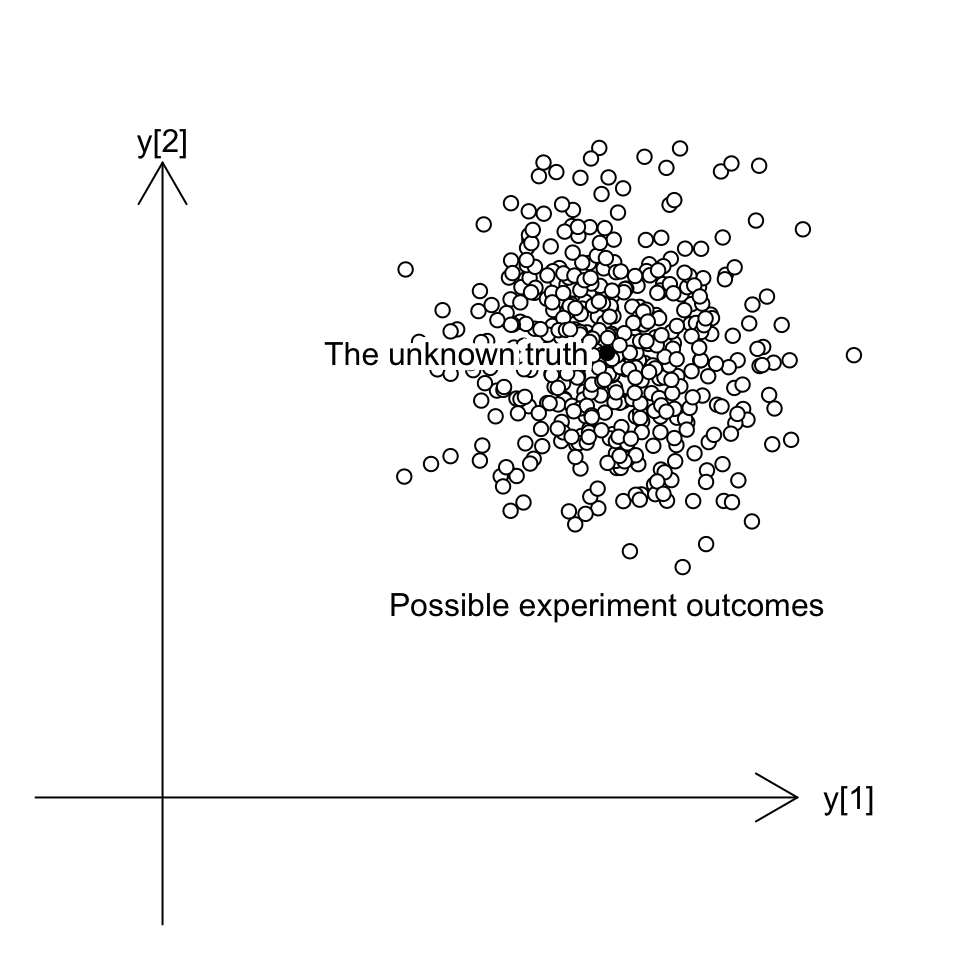## Model fitting – estimating $$\beta$$ with geometry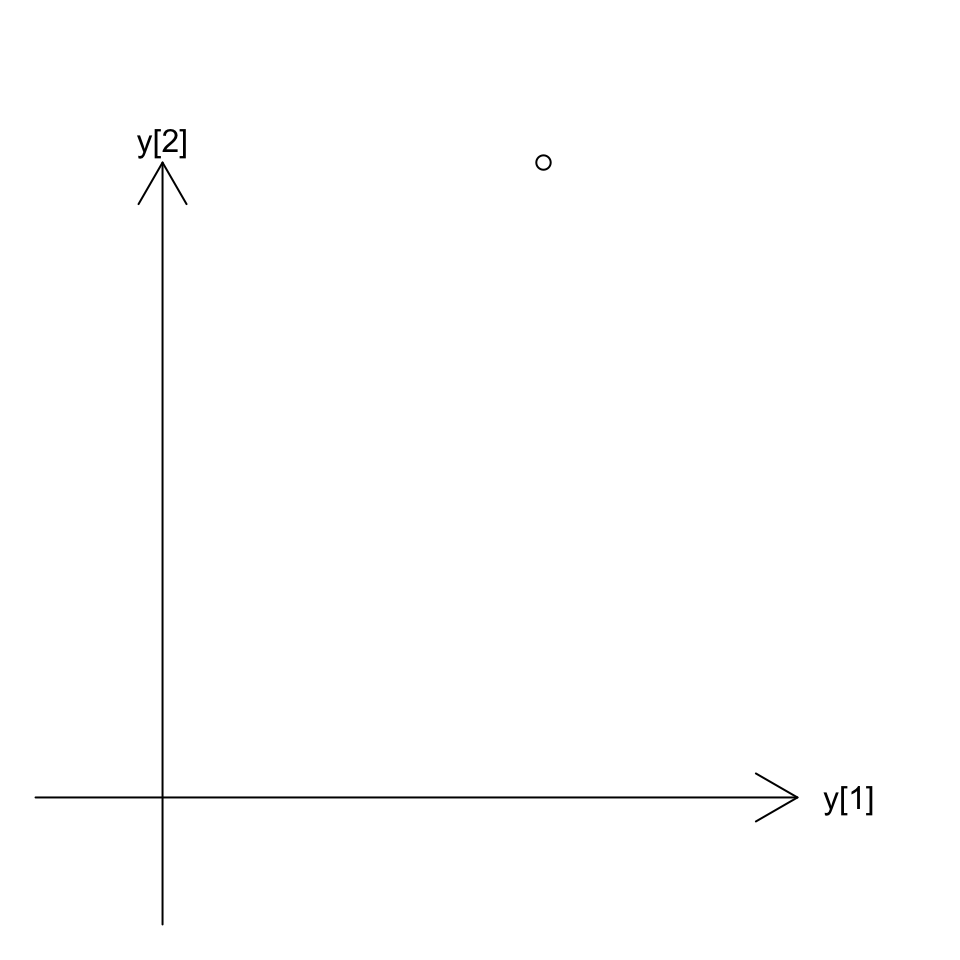## Model fitting – estimating $$\beta$$ with geometry

y <- c(3,5)
fit <- lm(y ~ 1)

coef(fit)
## (Intercept)
##           4
predict(fit)
## 1 2
## 4 4
residuals(fit)
##  1  2
## -1  1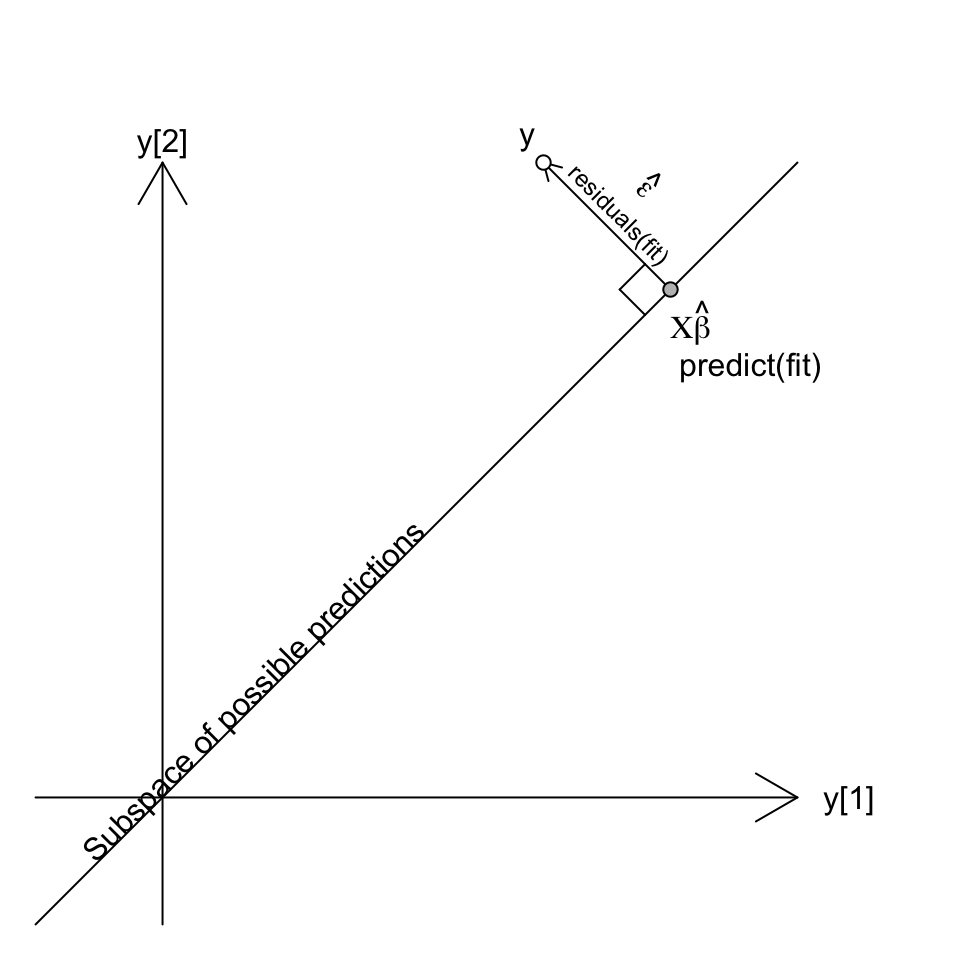## Model fitting – estimating $$\sigma$$

The coefficient is wrong, but is our best estimate. It is an unbiassed estimate.

The residuals vector must be orthogonal to the subspace of possible predictions so it is too short, but we can correct for this to get an unbiassed estimate of the variance. * In R, this is done using QR decomposition. X is decomposed into $$X=QR$$ with $$Q$$ orthonormal (i.e. a rotation matrix). $$Q^{\top}y$$ rotates $$y$$ so that the first $$p$$ elements contain all the information relevant to fitting the model, and the remaining $$n-p$$ elements are purely noise.

The first $$p$$ elements of $$Q^{\top}\hat{\varepsilon}$$ will be zero due to fitting the model, but the remaining $$n-p$$ elements are unaffected by this and as this is a rotation of a spherically symmetric noise distribution they have the same variance as the original elements of $$\varepsilon$$.

$\hat \sigma^2 = { \hat\varepsilon^\top \hat\varepsilon \over n-p }$

df.residual(fit)  # n-p
##  1
sigma(fit)
##  1.414214